# Mathematics All India Set 1 2015-2016 CBSE (English Medium) Class 10 Question Paper Solution

Mathematics [All India Set 1]
Date & Time: 19th March 2016, 10:30 am
Duration: 3h

 1

In Fig. 1, PQ is a tangent at a point C to a circle with centre O. if AB is a diameter and ∠CAB = 30°, find ∠PCA.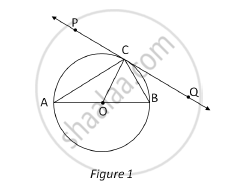Concept: Tangent to a Circle
Chapter: [3.01] Circles
 2

For what value of k will k + 9, 2k – 1 and 2k + 7 are the consecutive terms of an A.P?

Concept: Arithmetic Progression
Chapter: [2.02] Arithmetic Progressions
 3

A ladder leaning against a wall makes an angle of 60° with the horizontal. If the foot of the ladder is 2.5 m away from the wall, find the length of the ladder

Concept: Right-angled Triangles and Pythagoras Property
Chapter: [3.02] Triangles
 4

A card is drawn at random from a well shuffled pack of 52 playing cards. Find the probability of getting neither a red card nor a queen.

Concept: Basic Ideas of Probability
Chapter: [5.01] Probability [5.01] Probability
 5

If -5 is a root of the quadratic equation 2x2 + px – 15 = 0 and the quadratic equation p(x2 + x)k = 0 has equal roots, find the value of k.

Concept: Nature of Roots
 6

Let P and Q be the points of trisection of the line segment joining the points A(2, -2) and B(-7, 4) such that P is nearer to A. Find the coordinates of P and Q.

Concept: Section Formula
Chapter: [6.01] Lines (In Two-dimensions)
 7

In the following Fig, a quadrilateral ABCD is drawn to circumscribe a circle, with centre O, in such a way that the sides AB, BC, CD and DA touch the circle at the points P, Q, R and S respectively. Prove that AB + CD = BC + DA.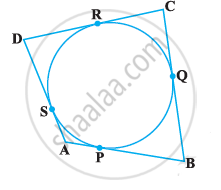Concept: Number of Tangents from a Point on a Circle
Chapter: [3.01] Circles
 8

Prove that the points (3, 0), (6, 4) and (-1, 3) are the vertices of a right-angled isosceles triangle.

Concept: Coordinate Geometry
Chapter: [6.01] Lines (In Two-dimensions) [6.01] Lines (In Two-dimensions)
 9

The 4th term of an A.P. is zero. Prove that the 25th term of the A.P. is three times its 11th term

Concept: Arithmetic Progression
Chapter: [2.02] Arithmetic Progressions
 10

In Fig.3, from an external point P, two tangents PT and PS are drawn to a circle with centre O and radius r. If OP = 2r, show that ∠ OTS = ∠ OST = 30°.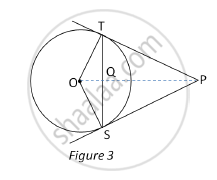Concept: Number of Tangents from a Point on a Circle
Chapter: [3.01] Circles
 11

In Fig. 4, O is the centre of a circle such that diameter AB = 13 cm and AC = 12 cm. BC is joined. Find the area of the shaded region. (Take π = 3.14)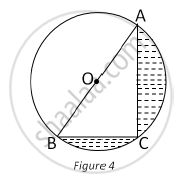Concept: Circumference of a Circle
Chapter: [7.01] Areas Related to Circles [7.01] Areas Related to Circles
 12

In Fig. 5, a tent is in the shape of a cylinder surmounted by a conical top of same diameter. If the height and diameter of cylindrical part are 2.1 m and 3 m respectively and the slant height of conical part is 2.8 m, find the cost of canvas needed to make the tent if the canvas is available at the rate of Rs. 500/sq. metre ( "Use "pi=22/7)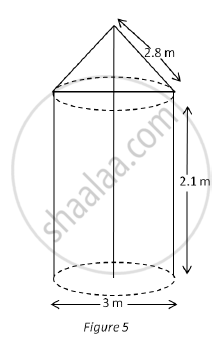Concept: Concept of Surface Area, Volume, and Capacity
Chapter: [7.02] Surface Areas and Volumes [7.02] Surface Areas and Volumes
 13

If the point P(x, y) is equidistant from the points A(a + b, b – a) and B(a – b, a + b). Prove that bx = ay.

Concept: Distance Formula
Chapter: [6.01] Lines (In Two-dimensions)
 14

In Fig. 6, find the area of the shaded region, enclosed between two concentric circles of radii 7 cm and 14 cm where ∠AOC = 40°. (use pi =  22/7)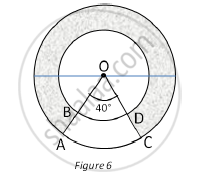Concept: Circumference of a Circle
Chapter: [7.01] Areas Related to Circles [7.01] Areas Related to Circles
 15

If the ratio of the sum of first n terms of two A.P’s is (7n +1): (4n + 27), find the ratio of their mth terms.

Concept: Sum of First n Terms of an AP
Chapter: [2.02] Arithmetic Progressions
 16

Solve for x

:1/((x-1)(x-2))+1/((x-2)(x-3))=2/3 , x ≠ 1,2,3

Concept: Solutions of Quadratic Equations by Factorization
 17

A conical vessel, with base radius 5 cm and height 24 cm, is full of water. This water is emptied into a cylindrical vessel of base radius 10 cm. Find the height to which the water will rise in the cylindrical vessel. (use pi=22/7)

Concept: Concept of Surface Area, Volume, and Capacity
Chapter: [7.02] Surface Areas and Volumes [7.02] Surface Areas and Volumes
 18

A sphere of diameter 12 cm, is dropped in a right circular cylindrical vessel, partly filled with water. If the sphere is completely submerged in water, the water level in the cylindrical vessel rises by 3 5/9 cm. Find the diameter of the cylindrical vessel.

Concept: Concept of Surface Area, Volume, and Capacity
Chapter: [7.02] Surface Areas and Volumes [7.02] Surface Areas and Volumes
 19

A man standing on the deck of a ship, which is 10 m above water level, observes the angle of elevation of the top of a hill as 60° and the angle of depression of the base of a hill as 30°. Find the distance of the hill from the ship and the height of the hill

Concept: Heights and Distances
Chapter: [4.01] Heights and Distances
 20

Three different coins are tossed together. Find the probability of getting exactly two heads.

Concept: Basic Ideas of Probability
Chapter: [5.01] Probability [5.01] Probability

Three different coins are tossed together. Find the probability of getting at least two heads.

Concept: Basic Ideas of Probability
Chapter: [5.01] Probability [5.01] Probability

Three different coins are tossed together. Find the probability of getting at least two tails.

Concept: Basic Ideas of Probability
Chapter: [5.01] Probability [5.01] Probability
 21

Due to heavy floods in a state, thousands were rendered homeless. 50 schools collectively offered to the state government to provide place and the canvas for 1500 tents to be fixed by the governments and decided to share the whole expenditure equally. The lower part of each tent is cylindrical of base radius 2.8 cm and height 3.5 m, with conical upper part of same base radius but of height 2.1 m. If the canvas used to make the tents costs Rs. 120 per sq. m, find the amount shared by each school to set up the tents. What value is generated by the above problem? (use pi =22/7)

Concept: Concept of Surface Area, Volume, and Capacity
Chapter: [7.02] Surface Areas and Volumes [7.02] Surface Areas and Volumes
 22

Prove that the lengths of the tangents drawn from an external point to a circle are equal.

Concept: Number of Tangents from a Point on a Circle
Chapter: [3.01] Circles
 23

Draw a circle of radius 4 cm. Draw two tangents to the circle inclined at an angle of 60° to each other.

Concept: Construction of Tangents to a Circle
Chapter: [3.03] Constructions
 24

In Fig. 7, two equal circles, with centres O and O’, touch each other at X. OO’ produced meets the circle with centre O’ at A. AC is tangent to the circle with centre O, at the point C. O’D is perpendicular to AC. Find the value of (DO')/(CO')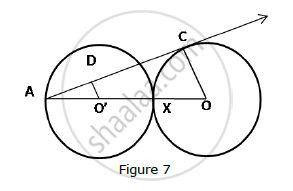Concept: Tangent to a Circle
Chapter: [3.01] Circles
 25

Solve for x: 1/(x+1)+2/(x+2)=4/(x+4), x ≠ -1, -2, -3

Concept: Nature of Roots
 26

The angle of elevation of the top Q of a vertical tower PQ from a point X on the ground is 60°. From a point Y, 40 m vertically above X, the angle of elevation of the top Q of tower is 45. Find the height of the tower PQ and the distance PX. (Use sqrt3=1.73)

Concept: Heights and Distances
Chapter: [4.01] Heights and Distances
 27

The houses in a row numbered consecutively from 1 to 49. Show that there exists a value of X such that sum of numbers of houses preceding the house numbered X is equal to sum of the numbers of houses following X.

Concept: Sum of First n Terms of an AP
Chapter: [2.02] Arithmetic Progressions
 28

In Fig. 8, the vertices of ΔABC are A(4, 6), B(1, 5) and C(7, 2). A line-segment DE is drawn to intersect the sides AB and AC at D and E respectively such that (AD)/(AB)=(AE)/(AC)=1/3 Calculate th area of ADE and compare it with area of ΔABCe.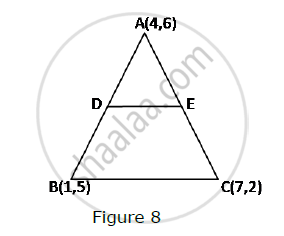Concept: Area of a Triangle
Chapter: [6.01] Lines (In Two-dimensions)
 29

A number x is selected at random from the numbers 1, 2, 3, and 4. Another number y is selected at random from the numbers 1, 4, 9 and 16. Find the probability that product of x and y is less than 16.

Concept: Basic Ideas of Probability
Chapter: [5.01] Probability [5.01] Probability
 30

In Fig. 9, is shown a sector OAP of a circle with centre O, containing ∠θ. AB is perpendicular to the radius OQ and meets OP produced at B. Prove that the perimeter of shaded region is

r[tantheta+sectheta+(pitheta)/180-1]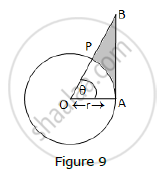Concept: Circumference of a Circle
Chapter: [7.01] Areas Related to Circles [7.01] Areas Related to Circles
 31

A motor boat whose speed is 24 km/h in still water takes 1 hour more to go 32 km upstream than to return downstream to the same spot. Find the speed of the stream.

Concept: Pair of Linear Equations in Two Variables
Chapter: [2.01] Pair of Linear Equations in Two Variables

#### Request Question Paper

If you dont find a question paper, kindly write to us

View All Requests

#### Submit Question Paper

Help us maintain new question papers on Shaalaa.com, so we can continue to help students

only jpg, png and pdf files

## CBSE previous year question papers Class 10 Mathematics with solutions 2015 - 2016

CBSE Class 10 Maths question paper solution is key to score more marks in final exams. Students who have used our past year paper solution have significantly improved in speed and boosted their confidence to solve any question in the examination. Our CBSE Class 10 Maths question paper 2016 serve as a catalyst to prepare for your Mathematics board examination.
Previous year Question paper for CBSE Class 10 Maths-2016 is solved by experts. Solved question papers gives you the chance to check yourself after your mock test.
By referring the question paper Solutions for Mathematics, you can scale your preparation level and work on your weak areas. It will also help the candidates in developing the time-management skills. Practice makes perfect, and there is no better way to practice than to attempt previous year question paper solutions of CBSE Class 10.

How CBSE Class 10 Question Paper solutions Help Students ?
• Question paper solutions for Mathematics will helps students to prepare for exam.
• Question paper with answer will boost students confidence in exam time and also give you an idea About the important questions and topics to be prepared for the board exam.
• For finding solution of question papers no need to refer so multiple sources like textbook or guides.